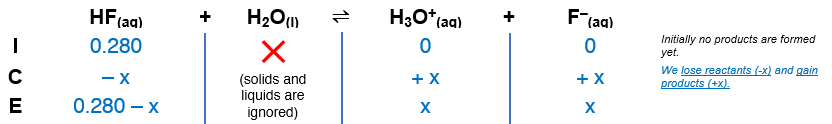# Problem: Determine the pH of HF solution of each of the following concentrations.a. 0.280 Mb. 5.00 x 10 -2 M

###### FREE Expert Solution

The dissociation of HF is as follows:

HF(aq) + H2O(l)  H3O+(aq) + F(aq); Ka = 6.6 × 10–4

The Ka expression for HF is:

$\overline{){{\mathbf{K}}}_{{\mathbf{a}}}{\mathbf{=}}\frac{\mathbf{products}}{\mathbf{reactant}}{\mathbf{=}}\frac{\left[{H}_{3}{O}^{+}\right]\left[{F}^{-}\right]}{\left[\mathrm{HF}\right]}}$

A. For  0.280 M:

Construct an ICE table.83% (156 ratings)###### Problem Details

Determine the pH of HF solution of each of the following concentrations.

a. 0.280 M

b. 5.00 x 10 -2 M